﻿ bgods - ggplot2学习笔记之构建图层

# ggplot2学习笔记之构建图层

## 创建绘图对象

``````ggplot(data, aes(x, y, <other aesthetics>))
``````

• 数据和图形属性映射；
• 一种统计变换；
• 一种几何对象；
• 一种位置调整方式。

``````geom_XXX(mapping, data, …, stat, position)
stat_XXX(mapping, data, …, geom, position)
``````
• mapping（可选）：一组图形属性映射，通过aes()函数来设定；
• data（可选）：数据框。如果不设定该参数，则会调用绘图对象中的默认数据集。
• 注意：在绘图对象ggplot()与图层中，mapping 与data 的位置是相反的；
• ：geom 或者 stat 的参数。可以是用图形属性作为参数，这样该属性就被设定为固定的一个值，而不是被映射给数据集中的一个变量；

• geom 或stat（可选）：我们可以修改geom 默认的stat 值，或者stat 默认的geom值。它们是一组字符串，包含了将要使用的几何对象或者统计变换的名称。

• position（可选）：选择一种调整对象重合的方式。

## 数据与图层映射

• data 是数据集，数据类型必须是数据框，可选；
• aes()函数用来将数据变量映射到图形中，从而使变量成为可以被感知的图形属性；
• x、y 是映射到x、y 轴上的变量。

``````p <- ggplot(mtcars, aes(x=mpg,y=wt))
``````

p + aes(colour = cyl) 添加colour 属性映射
p + aes(y = disp) 修改y 轴映射属性
p + aes(y = NULL) 删除 y 轴的映射属性

``````p <- ggplot(dsmall, aes(carat, price))
p + geom_point()
``````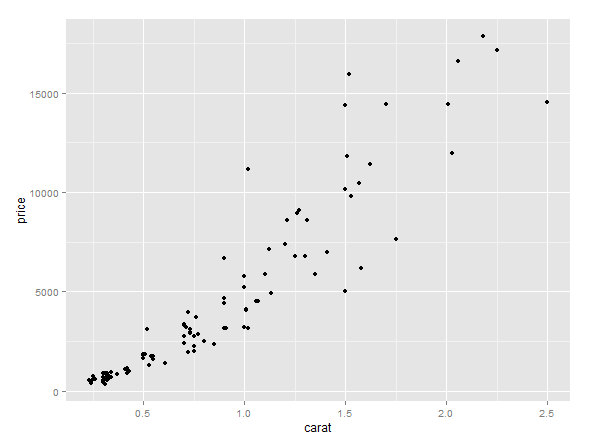``````p + geom_point() + geom_smooth()
``````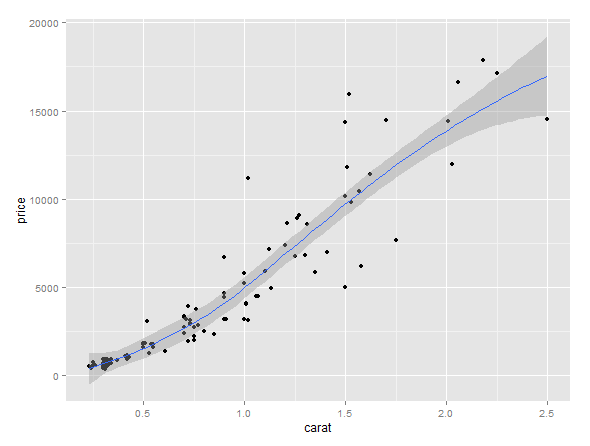``````ggplot(dsmall, aes(x = carat, y = price, colour = cut)) + geom_line()
``````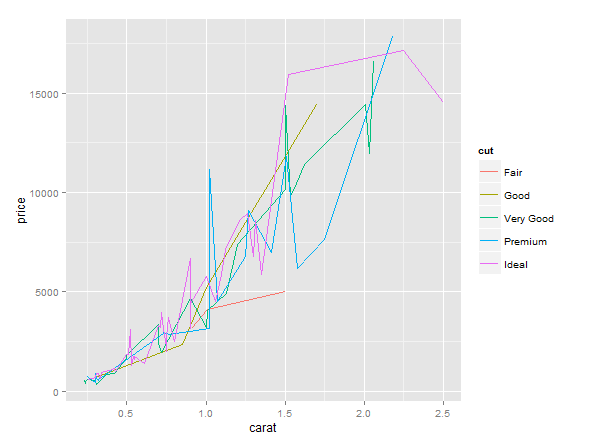``````p <- ggplot(mtcars, aes(mpg, wt))
p + geom_point(aes(colour = 'red'))
``````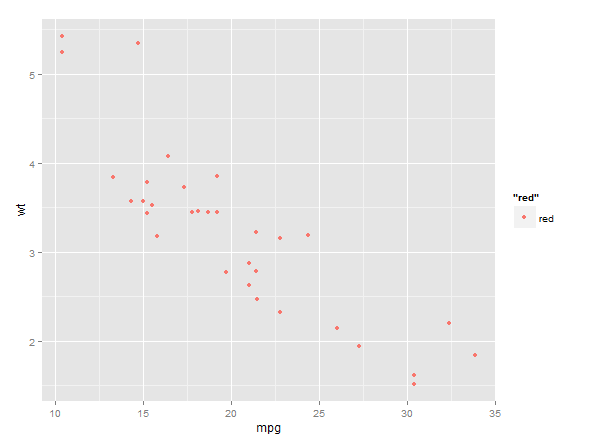``````ggplot(dsmall, aes(carat, price, group = cut)) + geom_line()
``````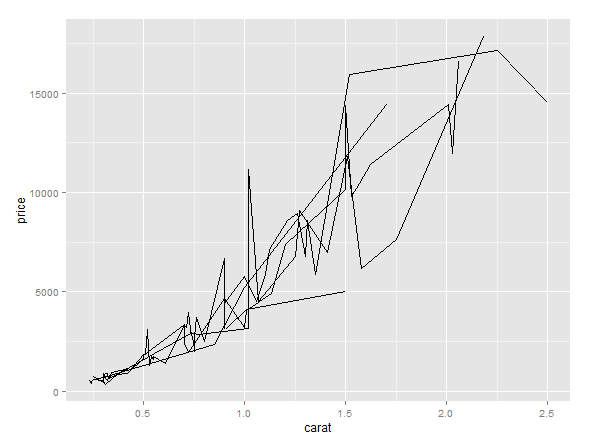``````ggplot(dsmall, aes(carat, price, colour = cut)) + geom_line()
````````````ggplot(dsmall, aes(carat, price, group = interaction(cut, color))) + geom_line()
``````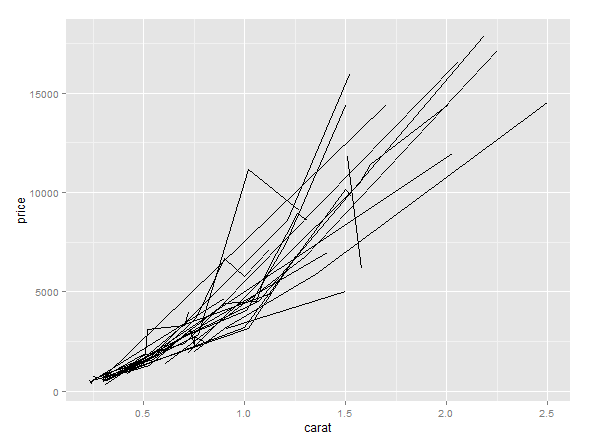## 几何对象

abline 线，由斜率与截距决定
area 面积图（area plot）
bar 条形图，以 x 轴为底的矩形
bin2d 二维热图
blank 空白，什么也不画
boxplot 箱线图
contour 等高线图
crossbar 带有水平中心线的盒子图
density 光滑密度曲线图
density2d 二维密度等高线图
dotplot “点直方图”，用点来表示观测值的个数
errorbar 误差棒
errorbarh 水平的误差棒
freqpoly 频率多边形图
hex 用六边形表示的二维热图
histogram 直方图
hline 水平线
jitter 给点添加扰动，减轻图形重叠问题
line 按照 x 坐标的大小顺序依次连接各个观测值
linerange 一条代表一个区间的竖直线
map 基准地图里的多边形
path 按数据的原始顺序连接各个观测值
point 点，绘制散点图
pointrange 用一条中间带点的竖直线代表一个区间
polygon 多边形，相当于一个有填充的路径
quantile 添加分位数回归线
raster 高效的矩形瓦片图
rect 二维的矩形图
ribbon 色带图，连续的 x 值所对应的 y 的范围
rug 边际地毯图
segment 添加线段或箭头
smooth 添加光滑的条件均值线
step 以阶梯形式连接各个观测值
text 文本注释
tile 瓦片图
violin 小提琴图
vline 竖直图

abline abline colour, linetype, size
area identity colour, fill, linetype, size, x, y
bar bin colour, fill, linetype, size, weight, x
bin2d bin2d colour, fill, linetype, size, weight, xmax, xmin, ymax, ymin
blank identity
boxplot boxplot colour, fill, lower, middle,size, upper, weight, x, ymax,ymin
contour contour colour, linetype, size, weight, x, y
crossbar identity colour, fill, linetype, size, x, y, ymax, ymin
density density colour, linetype, size, weight, x, y
density2d density2d colour, linetype, size, weight, x, y
dotplot bindot colour, fill, x, y
errorbar identity colour, linetype, size, width, x, ymax, ymin
errorbarh identity colour, linetype, size, width, x, ymax, ymin
freqpoly bin colour, linetype, size
hex binhex colour, fill, size, x, y
histogram bin colour, fill, linetype, size, weight, x
hline hline colour, linetype, size
jitter identity colour, fill, shape, size, x, y
line identity colour, linetype, size, x, y
linerange identity colour, linetype, size, x, ymax, ymin
map identity colour, fill, linetype, size, x, y, map_id
path identity colour, linetype, size, x, y
point identity colour, fill, shape, size, x, y
pointrange identity colour, fill, linetype, shape, size, x, y, ymax, ymin
polygon identity colour, fill, linetype, size, x, y
quantile quantile colour, linetype, size, weight, x, y
raster identity colour, fill, linetype, size, x, y
rect identity colour, fill, linetype, size, xmax, xmin, ymax, ymin
ribbon identity colour, fill, linetype, size, x, ymax, ymin
rug identity colour, linetype, size
segment identity colour, linetype, size, x, xend, y, yend
smooth smooth alpha, colour, fill, linetype, size, weight, x, y
step identity colour, linetype, size, x, y
text identity angle, colour, hjust, label, size, vjust, x, y
tile identity colour, fill, linetype, size, x, y
violin ydensity weight, colour, fill, size, linetype, x, y
vline vline colour, linetype, size

## 统计变换

bin 计算封箱（bin）数据
bin2d 计算矩形封箱内的观测值个数
bindot 计算“点直方图”的封箱数据
binhex 计算六边形热图的封箱数据
boxplot 计算组成箱线图的各种元素值
contour 三维数据的等高线
density 一维密度估计
density2d 二维密度估计
function 添加新函数
identity 不对数据进行统计变换
qq 计算 qq 图的相关值
quantile 计算连续的分位数
smooth 添加光滑曲线
spoke 将角度和半径转换成 xend 和yend
sum 计算每个单一值的频数，有助于解决散点图的图形重叠问题
summary 对每个 x 所对应的 y 值做统计描述
summary2d 对 2 维矩形封箱设定函数
summaryhex 对 2 维六边形封箱设定函数
unique 删除重复值
ydensity 小提琴图，计算 1 维y 轴方向的核密度函数估计值

• count，每一组观测值数目；
• density，每组观测值的密度（占整体百分数/组宽）；
• x，组的中心位置。

``````ggplot(diamonds, aes(carat)) + stat_bin(binwidth = 0.1)
``````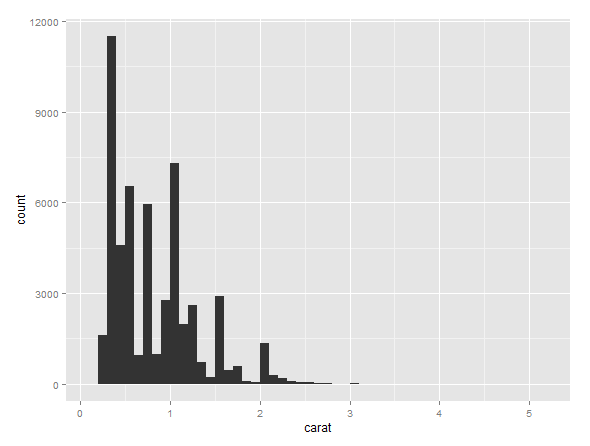``````ggplot(diamonds, aes(carat)) + stat_bin(aes(y = ..density..), binwidth = 0.1)
``````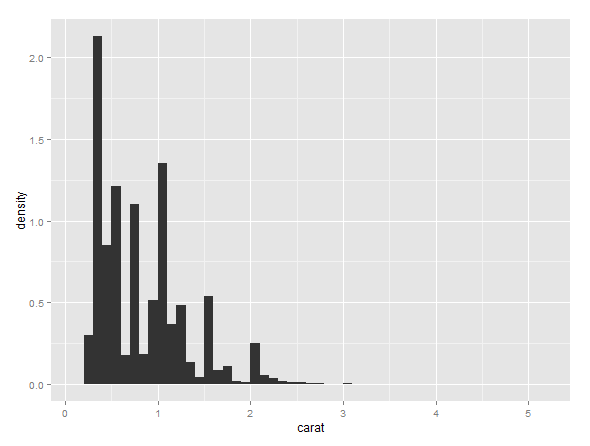## 位置调整

dodge 避免重叠，并排位置
fill 堆叠图形元素并将高度标准化为 1
identity 不做任何调整
jitter 给点添加扰动避免重合
stack 将图形元素堆叠起来

``````ggplot(diamonds, aes(clarity, fill = cut)) + geom_bar(position = 'stack')
ggplot(diamonds, aes(clarity, fill = cut)) + geom_bar(position = 'fill')
ggplot(diamonds, aes(clarity, fill = cut)) + geom_bar(position = 'dodge')
``````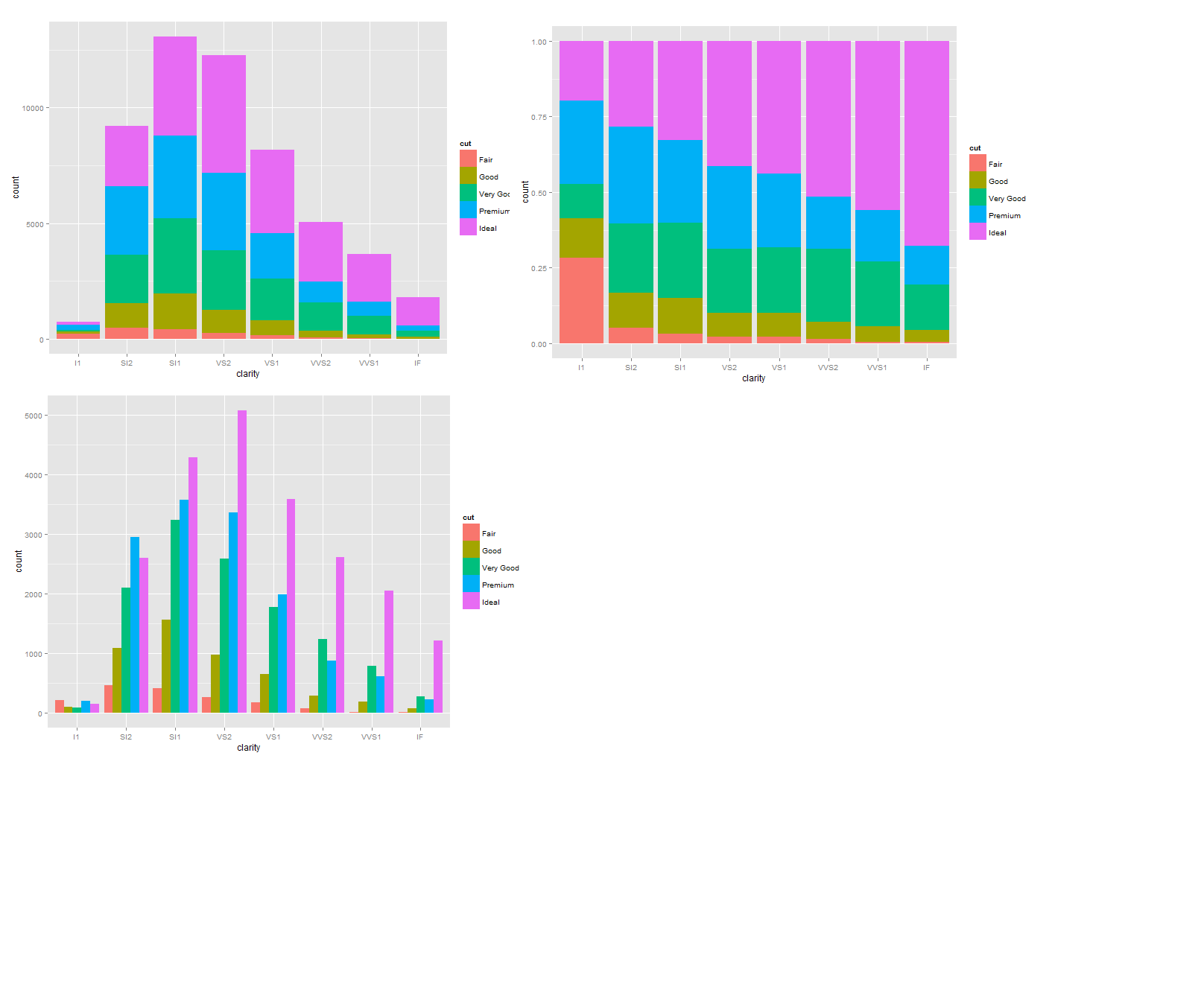``````ggplot(diamonds, aes(clarity, fill = cut)) + geom_bar(position = 'identity')
ggplot(diamonds, aes(clarity)) + geom_freqpoly(aes(group = cut, colour = cut))``````﻿

0 评论